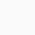# More Fun with Prime Numbers

Take a deep dive into Prime Numbers – one of the most mysterious and important subjects in mathematics!

2, 3, 5, 7, 11, 13, 17, 19, 23, 29 are all prime numbers and they hold special significance. Mathematicians from ancient times to the 21st century have been working on prime numbers, as they’re one of the most mysterious and important subjects in mathematics.

In this course, I will present several attractive topics on prime numbers. You will learn basic concepts of prime numbers from the beginning. They obey mysterious laws. Some laws are easily verified by hand, some laws were discovered 100 years ago, and some laws are yet to be discovered. Surprisingly, prime numbers are also applied to cryptography today. You will also learn how to construct practical cryptosystems using prime numbers.

The original course “Fun with Prime Numbers” was first offered in 2015 and attracted many students. This course will be offered as its refined and upgraded version. All the lecture videos will be renewed, and a new topic on cryptography will be added so as to enliven and satisfy even the students who took the previous course.

No previous knowledge of prime numbers is required in this course. Calculating with a pen and paper, you will explore the mysterious world of prime numbers. The course is designed to encourage you to attack unsolved problems, and hopefully, discover new laws of your own in the future!

• Basic Properties of Prime Numbers
• Modular Arithmetic and Fermat’s Little Theorem
• Laws of Prime Numbers
• Applications of Prime Numbers to Cryptography
• Open Problems and Recent Advances

Week1: What are Prime Numbers?
Introduction to basic concepts and properties of prime numbers, such as infinitude of prime numbers, counting prime numbers, and the Basel problem and its relation with the Riemann Hypothesis.

Week 2: Sums of Two Squares
Introduction to modular arithmetic and its applications to number theory, including Fermat’s Little Theorem, Wilson’s Theorem, and Fermat’s theorem on sums of two squares.

Week 3: The Reciprocity Laws
Introduction to the quadratic reciprocity laws proved by Gauss. Several generalizations of the quadratic reciprocity laws are also explained.

Week 4: Prime Numbers and Cryptography
Introduction to cryptography, and the construction of practical cryptosystems using prime numbers. More recent topics on elliptic curve cryptosystems are also explained.

Week 5: Mystery of Prime Numbers: Past, Present, and Future
Introduction to several open problems and conjectures on prime numbers, including the Birch and Swinnerton-Dyer conjecture and the ABC conjecture.

0

We will be happy to hear your thoughts